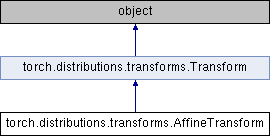Caffe2 - Python API A deep learning, cross platform ML framework
torch.distributions.transforms.AffineTransform Class Reference
Inheritance diagram for torch.distributions.transforms.AffineTransform:## Public Member Functions

def __init__ (self, loc, scale, event_dim=0, cache_size=0)

def __eq__ (self, other)

def sign (self)

def log_abs_det_jacobian (self, x, y)Public Member Functions inherited from torch.distributions.transforms.Transform
def __init__ (self, cache_size=0)

def inv (self)

def sign (self)

def __eq__ (self, other)

def __ne__ (self, other)

def __call__ (self, x)

def log_abs_det_jacobian (self, x, y)

def __repr__ (self)

loc

scale

event_dim

## Static Public Attributes

domain

codomain

bijectiveStatic Public Attributes inherited from torch.distributions.transforms.Transform
bijective

event_dim

## Detailed Description

Definition at line 380 of file transforms.py.

The documentation for this class was generated from the following file: